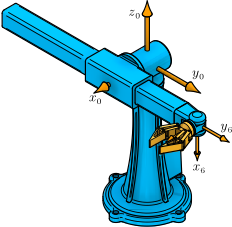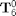# Example 3.7:: Offset Spherical Manipulator: Forward Kinematics (MATLAB)This example computes the forward kinematics for the offset spherical manipulator in a specific configuration.

## Clear All Workspace Objects and Reset All Assumptions

clear all


## Joint Values

theta1 = 90; % deg
theta2 = -90; % deg
d3 = 50; % cm
theta4 = 90; % deg
theta5 = 90; % deg
theta6 = -90; % deg


## Structural Parameters

d2 = 18; %cm


## Local Transformation Variables

U512 = -cosd(theta5)*sind(theta6);
U522 = -sind(theta5)*sind(theta6);
U412 = U512*cosd(theta4) - sind(theta4)*cosd(theta6);
U413 = -cosd(theta4)*sind(theta5);
U422 = U512*sind(theta4) + cosd(theta4)*cosd(theta6);
U423 = -sind(theta4)*sind(theta5);
U212 = U412*cosd(theta2) - U522*sind(theta2);
U213 = U413*cosd(theta2) - sind(theta2)*cosd(theta5);
U214 = -d3*sind(theta2);
U222 = U412*sind(theta2) + U522*cosd(theta2);
U223 = U413*sind(theta2) + cosd(theta2)*cosd(theta5);
U224 = d3*cosd(theta2);


## The 2nd Column ofU112 = U212*cosd(theta1) - U422*sind(theta1);
U122 = U212*sind(theta1) + U422*cosd(theta1);
j6Res0 = [U112; U122; U222];


## The 3rd Column ofU113 = U213*cosd(theta1) - U423*sind(theta1);
U123 = U213*sind(theta1) + U423*cosd(theta1);
k6Res0 = [U113; U123; U223];


## The 1st Column ofi6Res0 = cross(j6Res0, k6Res0);


## The 4th Column ofU114 = U214*cosd(theta1) + d2*sind(theta1);
U124 = U214*sind(theta1) - d2*cosd(theta1);
X6Rel0Res0 = [U114; U124; U224];


## The Forward Kinematics as the Homogeneous TransformationT60 = [i6Res0 j6Res0 k6Res0 X6Rel0Res0;
0      0      0      1]

T60 =

0     0     1    18
0     1     0    50
-1     0     0     0
0     0     0     1



This MATLAB example illustrates a computation from the textbook Fundamentals of Robot Mechanics by G. L. Long, Quintus-Hyperion Press, 2015. See http://www.RobotMechanicsControl.info for additional relevant files.